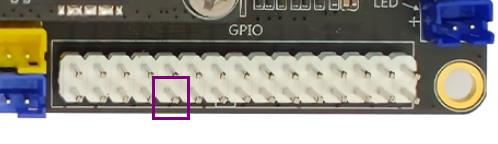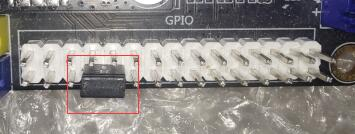# ¶ GPIO

GPIO(General Purpose Input/Output), which is a general-purpose pin that can be dynamically configured and controlled during software operation. On the RK platform, except for some dedicated pins (such as DDR, MIPI, etc.), the default multiplexing state for all pins is GPIO if multiplexing is not configured. RK's GPIO driver provides a standard GPIO interface for Linux. Linux has a set of sysfs nodes that operate GPIO in user mode. The application layer mainly implements GPIO programming by operating these nodes.The following is an example of operating GPIO3_A5. The location of this GPIO is as follows"The nodes under"/sys/class/gpio "require root permission to operate. Using command line or C language programs to operate gpio requires root permission.". If you are using SSH or an LX terminal, first execute the following command to obtain root privileges.

``````sudo su
``````

Under the generic Linux framework, all GPIOs are numbered numerically. For chips on the RK platform, the numbering calculation method is

(gpio controller number - 0) * 32 (port number - 'A') * 8 + (index number)

The controller number of GPIO3_A5 is 3, the port number is A, and the index number is 5, so the number is
(3-0)32+08+5=101

• Command line operation of GPIOs
• C language program to operate GPIO
• Set GPIO 101 to user state operation

``````echo 101 > /sys/class/gpio/export
``````

Set GPIO 101 to output

``````echo out > /sys/class/gpio/gpio101/direction
``````

In the case where GPIO 101 is an output, set the level

High level

``````echo 1 > /sys/class/gpio/gpio101/value
``````

Low level

``````echo 0 > /sys/class/gpio/gpio101/value
``````

Set GPIO 101 as input

``````echo in > /sys/class/gpio/gpio101/direction
``````

If GPIO 101 is an input, read its level, 1 is high, 0 is low

``````cat sys/class/gpio/gpio101/value
``````

Cancel GPIO 101 user state operation

``````echo 101 > /sys/class/gpio/unexport
``````

#### ¶ API Introduction

By linking to the libperipheral_api.a static library, the following interfaces can be called in C to operate GPIO

``````typedef enum {
PIN_DIRECTION_IN = 0,
PIN_DIRECTION_OUT = 1
} Enum_PinDirection;

/**
* @name: user_gpio_init
* @description: Configure a pin as gpio
* @param pin_num: pin num
* @return equal to 0 - Success; less than 0 - Failure
*/
int user_gpio_init(int pin_num);

/**
* @name: user_gpio_deinit
* @description: Release a pin
* @param pin_num: pin num
* @return equal to 0 - Success; less than 0 - Failure
*/
int user_gpio_deinit(int pin_num);

/**
* @name: user_gpio_set_level
* @description: Set gpio level
* @param pin_num: pin num
* @param level: 0 - low level; 1- high level
* @return equal to 0 - Success; less than 0 - Failure
*/
int user_gpio_set_level(int pin_num, unsigned char level);

/**
* @name: user_gpio_get_level
* @description: Get gpio level
* @param pin_num: pin num
* @param level: 0 - low level; 1- high level
* @return equal to 0 - Success; less than 0 - Failure
*/
int user_gpio_get_level(int pin_num, unsigned char *level);

/**
* @name: user_gpio_set_direction
* @description: Set gpio direction
* @param pin_num: pin num
* @param direction: PIN_DIRECTION_IN - input PIN_DIRECTION_OUT - output
* @return equal to 0 - Success; less than 0 - Failure
*/
int user_gpio_set_direction(int pin_num, unsigned char direction);

/**
* @name: user_gpio_get_direction
* @description: Get gpio direction
* @param pin_num: pin num
* @param direction: PIN_DIRECTION_IN - input PIN_DIRECTION_OUT - output
* @return equal to 0 - Success; less than 0 - Failure
*/
int user_gpio_get_direction(int pin_num, unsigned char *direction);
``````

#### ¶ demo

The test demo is as follows, taking GPIO0_D5 and GPIO3_A5 shown below as an example, shorting the two GPIOs, with the former set as output and the latter set as inputWiring is as follows``````void gpio_api_test(void)
{
unsigned char level = 0;
// GPIO0_D5 为29脚 GPIO3_A5 为101脚
user_gpio_init(29);
user_gpio_init(101);

user_gpio_set_direction(29, PIN_DIRECTION_OUT);
user_gpio_set_direction(101, PIN_DIRECTION_IN);

user_gpio_set_level(29, 1);
user_gpio_get_level(101, &level);

printf("1.pin 101 level %d\n", level);

usleep(10);

user_gpio_set_level(29, 0);
user_gpio_get_level(101, &level);

printf("2.pin 101 level %d\n", level);

user_gpio_deinit(29);
user_gpio_deinit(101);

return;
}

int main()
{

gpio_api_test();

return 0;
}
``````

The results of the run are as follows